I am working through the material in the Penn State online class STAT 501 Regression Methods. This R script is a personal exercise to translate the concepts and examples illustrated in Minitab into R and SAS. Lesson 2 in STAT 501 is SLR Model Evaluation. The SAS version of the code below is posted on my blog.

## Overview

There are three hypothesis tests for testing the existence of a linear relationship. For SLR, they always yield the same p-values.

• The t-test for $$H_0: \beta_1 = 0$$.

• The ANOVA F-test for $$H_0: \beta_1 = 0$$, and

• The t-test for $$H_0: \rho = 0$$.

The residuals in the SLR can be decomposed into their two causes: lack of fit in the mode, and random error. If a line fits the data well, the average of the observed responses at each x-value should be close to the predicted response for that x-value. Therefore, to determine how much of the total error is due to lack of model fit, the lack of fit F-test calculates the lack-of-fit sum of squares and a pure error sum of squares. The F-statistic for the lack of fit F-test is the ratio of the lack of fit mean square MSLF and the pure error mean squre. The null hypothesis is $$H_0:$$ no lack of fit.

## R Concepts Worth Noting

• The summary function does not provide a 95% confidence interval for the parameter estimators. Use the confint.lm function.

• The summary function does not provide an ANOVA table either. Use the anova function.

• Conduct a lack-of-fit test with the pureErrorAnova function.

## Data Management for this Module

library(readr)
library(ggplot2)
library(dplyr)
##
## Attaching package: 'dplyr'
## The following objects are masked from 'package:stats':
##
##     filter, lag
## The following objects are masked from 'package:base':
##
##     intersect, setdiff, setequal, union
library(alr3)
## Warning: package 'alr3' was built under R version 3.4.3
## Loading required package: car
## Warning: package 'car' was built under R version 3.4.3
##
## Attaching package: 'car'
## The following object is masked from 'package:dplyr':
##
##     recode
# skincancer is a fixed-width data file.
file="https://onlinecourses.science.psu.edu/stat501/sites/onlinecourses.science.psu.edu.stat501/files/data/skincancer.txt",
skip = 1,
fwf_widths(c(25, 9, 7, 5, 5)))
## Parsed with column specification:
## cols(
##   X1 = col_character(),
##   X2 = col_double(),
##   X3 = col_integer(),
##   X4 = col_integer(),
##   X5 = col_double()
## )
colnames(skincancer) <- c('state','lat','mort','ocean','lon')
# leadcord is a fixed-width data file.
skip = 1,
fwf_widths(c(4, 8, 7, 7)))
## Parsed with column specification:
## cols(
##   X1 = col_integer(),
##   X2 = col_integer(),
##   X3 = col_integer(),
##   X4 = col_double()
## )
colnames(leadcord) <- c('Month','Year','Sold','Cord')
# heightgpa is a tab-delimited data file.
url <- 'https://onlinecourses.science.psu.edu/stat501/sites/onlinecourses.science.psu.edu.stat501/files/data/heightgpa.txt'
heightgpa <- read.table(url, sep = '\t', header = TRUE)
# mens200m is a tab-delimited data file.
url <- 'https://onlinecourses.science.psu.edu/stat501/sites/onlinecourses.science.psu.edu.stat501/files/data/mens200m.txt'
mens200m <- read.table(url, sep = '\t', header = TRUE, skip = 1)
colnames(mens200m) <- c('Year', 'Men200m')
# husbandwife is a tab-delimited data file.
# Note the "*" in data represents null
url <- 'https://onlinecourses.science.psu.edu/stat501/sites/onlinecourses.science.psu.edu.stat501/files/data/husbandwife.txt'
husbandwife[husbandwife == "*"] <- NA
husbandwife <- transform(husbandwife, HAge = as.numeric(HAge))
husbandwife <- transform(husbandwife, HHght = as.numeric(HHght))
husbandwife <- transform(husbandwife, WAge = as.numeric(WAge))
husbandwife <- transform(husbandwife, WHght = as.numeric(WHght))
husbandwife <- transform(husbandwife, HAgeMar = as.numeric(HAgeMar))
# newaccounts is a tab-delimited data file.
url <- 'https://onlinecourses.science.psu.edu/stat501/sites/onlinecourses.science.psu.edu.stat501/files/data/newaccounts.txt'
newaccounts <- read.table(url, sep = '\t', header = TRUE)
# signdist is a tab-delimited data file.
url <- 'https://onlinecourses.science.psu.edu/stat501/sites/onlinecourses.science.psu.edu.stat501/files/examples/signdist.txt'
signdist <- read.table(url, sep = '\t', header = TRUE)
# handheight is a tab-delimited data file.
url <- 'https://onlinecourses.science.psu.edu/stat462/sites/onlinecourses.science.psu.edu.stat462/files/data/handheight.txt'
head(handheight)
##      Sex Height HandSpan
## 1 Female     68     21.5
## 2   Male     71     23.5
## 3   Male     73     22.5
## 4 Female     64     18.0
## 5   Male     68     23.5
## 6 Female     59     20.0

## 2.1 Inference for the Population Intercept and Slope

Revisit the example concerning the relationship between skin cancer mortality and state latitude (skincancer.txt). The response variable mort is the mortality rate (number of deaths per 10 million people) of white males due to malignant skin melanoma from 1950-1959. The predictor variable lat is the latitude (degrees North) at the center of each of 49 states in the United States. Here are the first few lines of data.

head(skincancer)
## # A tibble: 6 x 5
##   state         lat  mort ocean   lon
##   <chr>       <dbl> <int> <int> <dbl>
## 1 Alabama      33.0   219     1  87.0
## 2 Arizona      34.5   160     0 112
## 3 Arkansas     35.0   170     0  92.5
## 4 California   37.5   182     1 120
## 5 Colorado     39.0   149     0 106
## 6 Connecticut  41.8   159     1  72.8

The scatterplot and fit line show a linear relationship. The regression slope is -5.9776.

ggplot(skincancer, aes(y=mort, x=lat)) +
geom_point() +
geom_smooth(method = "lm") +
ylab("Mortality (Deaths per 10 million)") +
xlab("Latitude (at center of state)") +
ggtitle("Skin Cancer Mortality versus State Latitude")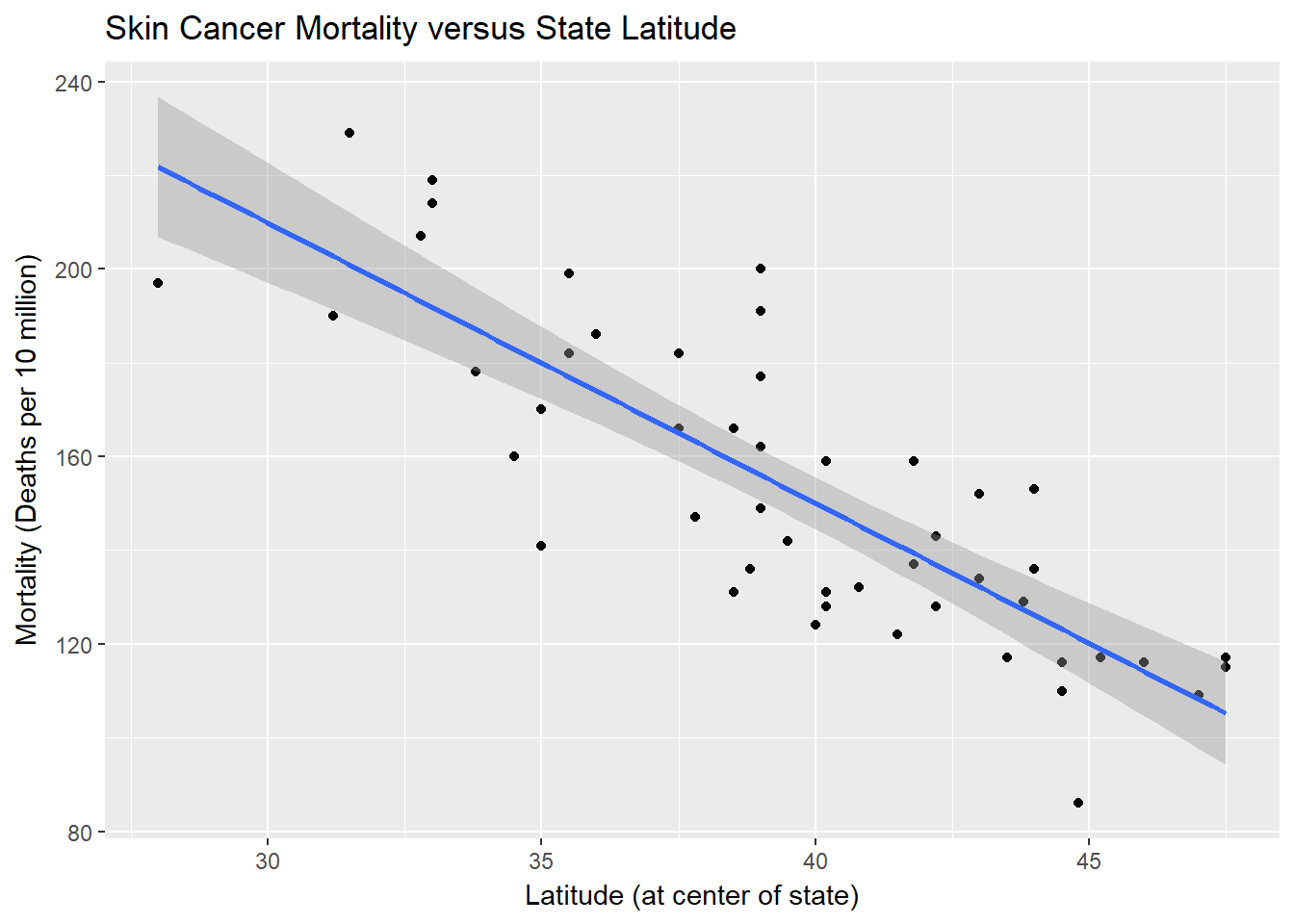summary(lm(mort ~ lat, data = skincancer))
##
## Call:
## lm(formula = mort ~ lat, data = skincancer)
##
## Residuals:
##     Min      1Q  Median      3Q     Max
## -38.972 -13.185   0.972  12.006  43.938
##
## Coefficients:
##             Estimate Std. Error t value Pr(>|t|)
## (Intercept) 389.1894    23.8123   16.34  < 2e-16 ***
## lat          -5.9776     0.5984   -9.99 3.31e-13 ***
## ---
## Signif. codes:  0 '***' 0.001 '**' 0.01 '*' 0.05 '.' 0.1 ' ' 1
##
## Residual standard error: 19.12 on 47 degrees of freedom
## Multiple R-squared:  0.6798, Adjusted R-squared:  0.673
## F-statistic:  99.8 on 1 and 47 DF,  p-value: 3.309e-13

The $$(1-\alpha)$$ confidence interval for the slope parameter $$\beta_1$$ is $$\beta_1 \pm t_(\alpha/2,n-2) \times se$$ where the standard error $$se = \sqrt MSE / \sum (x_i - \bar x)^2$$. In this case, $$\beta_1=-5.9776$$, and $$se=0.5984$$. The critical $$t_(\alpha/2,n-2)=2.012$$ (see code below).

qt(.975, df=47)
##  2.011741
The width of the confidence interval decreases…
• …as $$\alpha$$ increase
• …as the MSE decreases. The MSE is affected by how observations naturally vary around the estimated regression line (out of your control), and how well the regression line fits the data (in your control!).
• …with greater spread in the explantory values, $$\sum (x_i - \bar x)^2$$.
• …with increasing number of observations by adding degrees of freedom to t, and by increasing the $$\sum (x_i - \bar x)^2$$.

The $$\alpha$$-level of significance t-test of $$H_0: b_1=\beta$$ (where $$\beta$$ is typically 0) uses test statistic $$t=(b_1-\beta)/se(b_1)$$. In the example above, $$t=(-5.9776-0)/.5984=-9.99$$. The p-value is <.0001, so we reject $$H_0$$ at the $$\alpha=.05$$ level of significance.

Note that there are four possible outcomes of the t-test. If we fail to reject $$H_0$$, 1) there really is no linear relationship (although their may be a curvilinear relationship), or 2) we made a Type II error and there really is a linear relationshi. If we do reject $$H_0$$, 3) there really is a linear relationship, or 4) we made a Type I error and there really is not linear relationship (but a curvilinear relationship may still be better).

• ## 2.2 Another Example of Slope Inference

This example explores whether there a positive relationship between sales of leaded gasoline and mean lead concentrations in umbilical-cord blood of babies born at a major Boston hospital over 14 months in 1980-1981. Data set leadcord has response variable Cord measured in (µl/dl) and explanatory variable Sold measured in (in metric tons).

The scatterplot and fit line show a linear relationship. The regression slope is -5.9776.

ggplot(leadcord, aes(y=Cord, x=Sold)) +
geom_point() +
geom_smooth(method = "lm") +
xlab("Sales of Leaded Gasoline (metric tons)") +
ggtitle("Fitted Line Plot")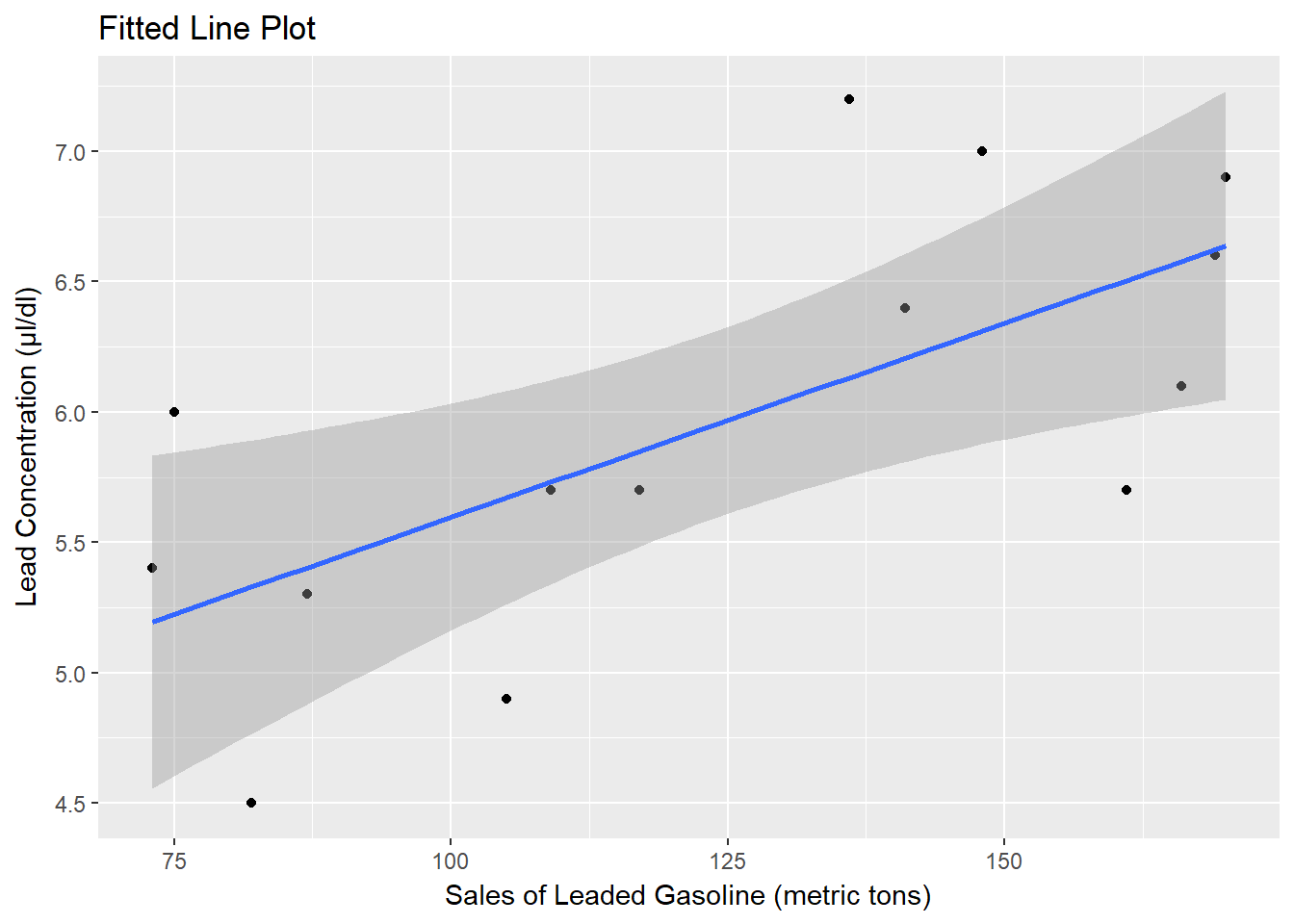m1<-lm(Cord ~ Sold, data = leadcord)
summary(m1)
##
## Call:
## lm(formula = Cord ~ Sold, data = leadcord)
##
## Residuals:
##      Min       1Q   Median       3Q      Max
## -0.82877 -0.39679 -0.02723  0.24729  1.06742
##
## Coefficients:
##             Estimate Std. Error t value Pr(>|t|)
## (Intercept) 4.108182   0.608806   6.748 2.05e-05 ***
## Sold        0.014885   0.004719   3.155   0.0083 **
## ---
## Signif. codes:  0 '***' 0.001 '**' 0.01 '*' 0.05 '.' 0.1 ' ' 1
##
## Residual standard error: 0.6162 on 12 degrees of freedom
## Multiple R-squared:  0.4533, Adjusted R-squared:  0.4078
## F-statistic: 9.952 on 1 and 12 DF,  p-value: 0.008303
confint.lm(m1, 2, level = .95)
##            2.5 %     97.5 %
## Sold 0.004604418 0.02516608

The P-value for Sold which tests $$H_0: \beta_1=0$$ is 0.0083. The P-value the one-sided hypothesis test $$H_0 : \beta_1 <= 0$$ is 0.008 ÷ 2 = 0.004, so there is sufficient statistical evidence at the $$\alpha=0.05$$ level to conclude $$\beta_1>0$$.

The 95% confidence interval for $$\beta_1$$ is (0.0046, 0.0252). Therefore we can be 95% confident that the mean lead concentrations in umbilical-cord blood of Massachusetts babies increases between 0.0046 and 0.0252 µl/dl for every one-metric ton increase in monthly gasoline lead sales in Massachusetts. Whether this is a meaningful increase is another matter.

## 2.3 Sums of Squares

The ANOVA table supports an alternative test of the linear relationship between the response variable and the explantory variable(s). Here is the ANOVA table from the skin cancer vs latitude example from earlier.

anova(lm(mort ~ lat, data = skincancer))
## Analysis of Variance Table
##
## Response: mort
##           Df Sum Sq Mean Sq F value    Pr(>F)
## lat        1  36464   36464  99.797 3.309e-13 ***
## Residuals 47  17173     365
## ---
## Signif. codes:  0 '***' 0.001 '**' 0.01 '*' 0.05 '.' 0.1 ' ' 1

The regression sum of squares (SSR) is the sum of the squared distances of predicted values on the fit line $$\hat y_i$$ to the “no-relationship” line, $$\bar y$$: $$SSR = \sum (\hat y_i - \bar y)^2 = 36464$$.

The total sum of squares (SST) is the sum of the squared distances of observed values $$y_i$$ to the “no-relationship” line, $$\bar y$$: $$SST = \sum (y_1 - \bar y)^2 = 53637$$ (not shown above). SST quantifies how much the observed responses vary if you do not take into account the explanatory variables.

The error sum of squares (SSE) is the sum of the squared distances of predicted values on the fit line $$\hat y_i$$ to the observed values, $$y_i$$: $$SSE = \sum (y_i - \hat y)^2 = 17173$$.

## 2.4 Sums of Squares (continued)

Consider again the GPA vs Student height example in which there is no statistically significant relationship in the t-test (p=.7613). The ANOVA table tells a similar story. $$SSR=.0276$$ while $$SSE=9.7055$$. The resuling R-squared is $$R-sq = SSR/(SSR+SSE) = .002835$$.

summary(lm(gpa ~ height, data = heightgpa))
##
## Call:
## lm(formula = gpa ~ height, data = heightgpa)
##
## Residuals:
##      Min       1Q   Median       3Q      Max
## -1.45081 -0.24878  0.00325  0.35622  0.90263
##
## Coefficients:
##              Estimate Std. Error t value Pr(>|t|)
## (Intercept)  3.410214   1.434616   2.377   0.0234 *
## height      -0.006563   0.021428  -0.306   0.7613
## ---
## Signif. codes:  0 '***' 0.001 '**' 0.01 '*' 0.05 '.' 0.1 ' ' 1
##
## Residual standard error: 0.5423 on 33 degrees of freedom
## Multiple R-squared:  0.002835,   Adjusted R-squared:  -0.02738
## F-statistic: 0.09381 on 1 and 33 DF,  p-value: 0.7613
anova(lm(gpa ~ height, data = heightgpa))
## Analysis of Variance Table
##
## Response: gpa
##           Df Sum Sq Mean Sq F value Pr(>F)
## height     1 0.0276 0.02759  0.0938 0.7613
## Residuals 33 9.7055 0.29411

## 2.5 Analysis of Variance: The Basic Idea

SST is the numerator of the sample variance. The sample variance is $$s^2 = SST / (n-1)$$ where $$n-1$$ is the degrees of freedom associated with SST. The mean square error MSE is $$MSE = SSE / (n-2)$$ where $$n-2$$ is the degrees of freedom associated with SSE.

## 2.6 The Analysis of Variance (ANOVA) table and the F-test

Imagine taking many random samples of size n from the population, estimating the regression line, and determining MSR and MSE for each sample. The expected value of the MSRs is $E(MSR) = \sigma^2 + \beta _1^2 \sum(X_i-\bar X)^2$. Similarly, the expected value of the MSEs is $E(MSE) = \sigma^2$.

If $$beta _1^2=0$$, then the ratio $$MSR/MSE=1$$. If $$beta _1^2 \ne 0$$, then the ratio $$MSR/MSE>1$$. The ratio of these two distributions follows an F distribution with $$1$$ numerator and $$n-2$$ denominator degrees of freedom.

## 2.7 Example: Are Men Getting Faster?

The data set mens200m contains the winning times (in seconds) of the 22 men’s 200 meter olympic sprints held between 1900 and 1996 (Men200m).

ggplot(mens200m, aes(y=Men200m, x=Year)) +
geom_point() +
geom_smooth(method = "lm") +
ylab("Winning Time (seconds)") +
xlab("Year") +
ggtitle("Fitted Line Plot")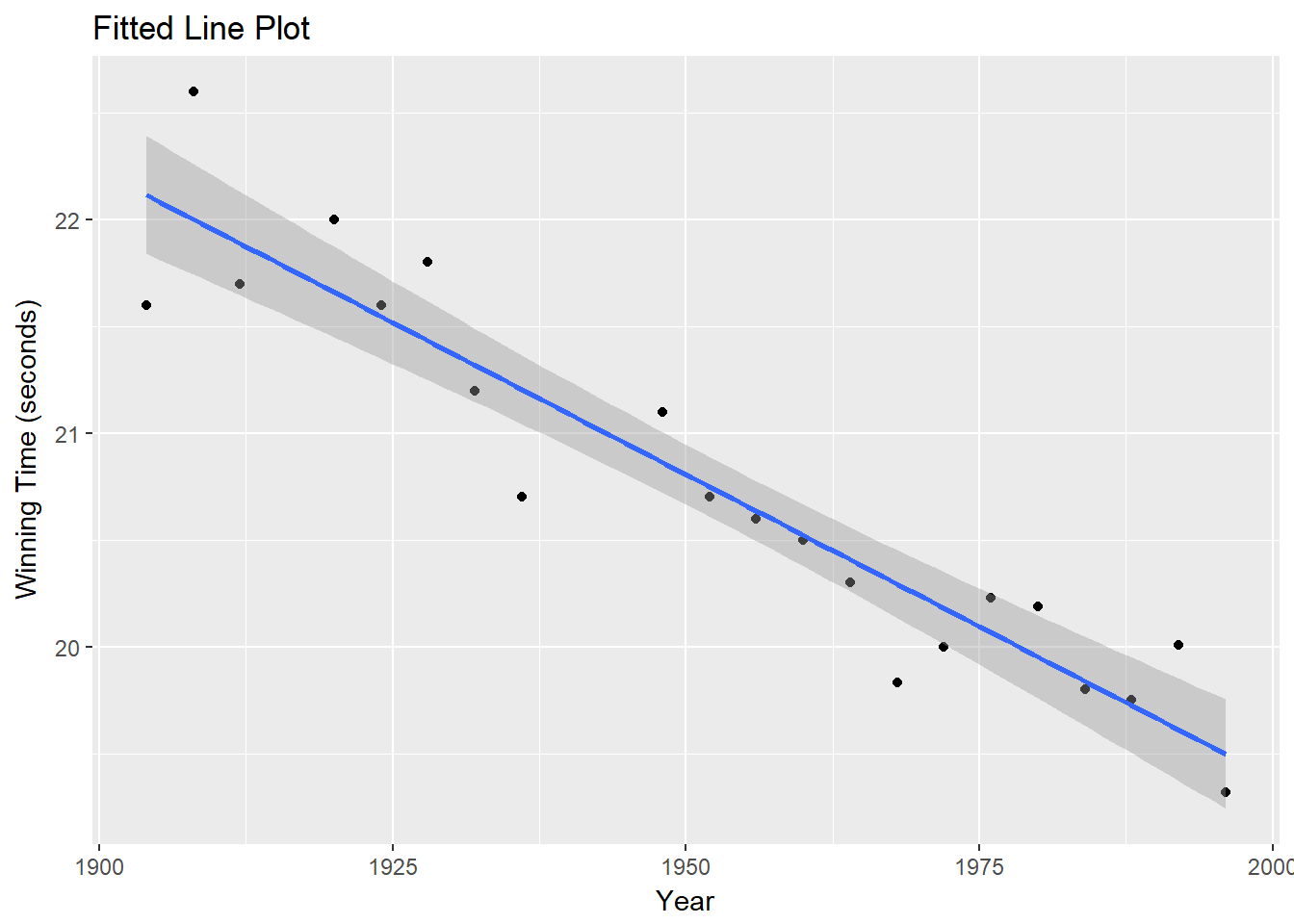Is there a linear relationship between year and the winning times? The parameter estimator for Year is $$-.028461$$ (p<.0001) meaning each successive year is associated with a winning time decreasing by .028461 seconds. The F-test reaches a similar conclusion. The ratio of MSR/MSE is $$F=147.1$$ (p<.0001).

summary(lm(Men200m ~ Year, data = mens200m))
##
## Call:
## lm(formula = Men200m ~ Year, data = mens200m)
##
## Residuals:
##      Min       1Q   Median       3Q      Max
## -0.51648 -0.17810 -0.03652  0.23579  0.59737
##
## Coefficients:
##              Estimate Std. Error t value Pr(>|t|)
## (Intercept) 76.305505   4.581259   16.66 8.61e-13 ***
## Year        -0.028461   0.002346  -12.13 2.16e-10 ***
## ---
## Signif. codes:  0 '***' 0.001 '**' 0.01 '*' 0.05 '.' 0.1 ' ' 1
##
## Residual standard error: 0.3058 on 19 degrees of freedom
## Multiple R-squared:  0.8856, Adjusted R-squared:  0.8796
## F-statistic: 147.1 on 1 and 19 DF,  p-value: 2.159e-10

Notice that in the case of the SLR where the F-statistic numerator degrees of freedom is 1, $$F=t^$$.

## 2.8 Equivalent Linear Relationship Tests

Note that the three hypothesis tests for testing the existence of a linear relationship always yield the same results.

• The t-test for $$H_0: \beta _1 = 0$$.

• The ANOVA F-test for $$H_0: \beta _1 = 0$$, and

• The t-test for $$H_0: \rho = 0$$.

In the example where we tested the association of the husband’s age with the wife’s age, we get t=34.975 (p<.001) for t-test for $$H_0: \beta _1 = 0$$; we get F=1223 (p<.0001) for the ANOVA F-test for $$H_0: \beta _1 = 0$$; and we get t = 34.975 for the t-test for $$H_0: \rho = 0$$.

summary(lm(HAge ~ WAge, data = husbandwife))
##
## Call:
## lm(formula = HAge ~ WAge, data = husbandwife)
##
## Residuals:
##      Min       1Q   Median       3Q      Max
## -13.8941  -1.9523  -0.8195   2.1179  17.1775
##
## Coefficients:
##             Estimate Std. Error t value Pr(>|t|)
## (Intercept)  1.73891    0.70778   2.457    0.015 *
## WAge         1.00597    0.02876  34.975   <2e-16 ***
## ---
## Signif. codes:  0 '***' 0.001 '**' 0.01 '*' 0.05 '.' 0.1 ' ' 1
##
## Residual standard error: 4.086 on 168 degrees of freedom
##   (48 observations deleted due to missingness)
## Multiple R-squared:  0.8792, Adjusted R-squared:  0.8785
## F-statistic:  1223 on 1 and 168 DF,  p-value: < 2.2e-16
cor.test(husbandwife$HAge, husbandwife$WAge, alternative = "two.sided")
##
##  Pearson's product-moment correlation
##
## data:  husbandwife$HAge and husbandwife$WAge
## t = 34.975, df = 168, p-value < 2.2e-16
## alternative hypothesis: true correlation is not equal to 0
## 95 percent confidence interval:
##  0.9165191 0.9536083
## sample estimates:
##      cor
## 0.937681
• ## 2.9 Notation for the Lack of Fit Test

The lack of fit F-test for linearity (a test of the linearity condition in the OLS model) requires repeat observations (replicates) for at least one of the values of the explanatory variable. In fact, we typically need quite a few for the test to have power.

Consider an a data set newaccounts with data for the size (Size) of the minimum deposit required when opening a new checking account at a bank and the number of new accounts at the bank (New). Suppose the trend in the data looks curved, but we fit a line anyway:

ggplot(newaccounts, aes(y=New, x=Size)) +
geom_point() +
geom_smooth(method = "lm") +
ylab("Number of New Accounts") +
xlab("Size of Minimum Deposit ($)") + ggtitle("Fitted Line Plot")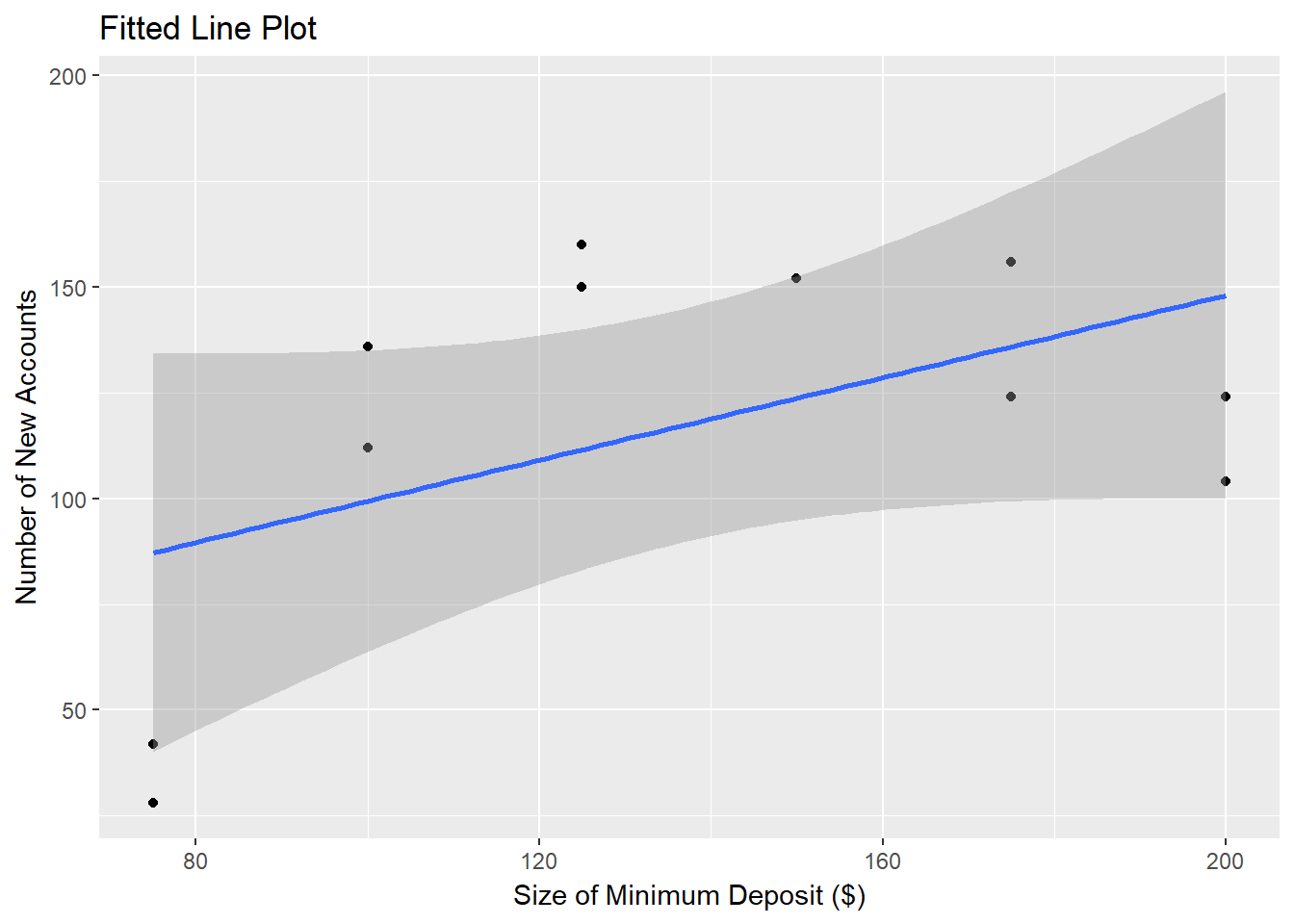Let $$y_ji$$ denote the j-th observation made at the i-th x-value in the data set. Let $$\bar y_i$$ denote the average observation value at the i-th x-value. Let $$\hat y_ij$$ denote the predicted value for the j-th observation made at the i-th x-value in the data set. ## 2.10 Decomposing the Error The residuals in the SLR can be decomposed into their two causes: lack of fit in the mode, and random error. If a line fits the data well, the average of the observed responses at each x-value should be close to the predicted response for that x-value. Therefore, to determine how much of the total error is due to lack of model fit, the lack of fit F-test calculates the lack-of-fit sum of squares $$\sum_ij (\bar y_i - \hat y_ij)^2$$ and a pure error sum of squares $$\sum_ij (y_ij - \hat y_ij)^2$$. In the checking account example most of the error (SSE = 14742) is attributable to the lack of a linear fit (SSLF = 13593.6) and not just to random error (SSPE = 1148). pureErrorAnova(lm(New ~ Size, data = newaccounts)) ## Analysis of Variance Table ## ## Response: New ## Df Sum Sq Mean Sq F value Pr(>F) ## Size 1 5141.3 5141.3 22.393 0.005186 ** ## Residuals 9 14741.6 1638.0 ## Lack of fit 4 13593.6 3398.4 14.801 0.005594 ** ## Pure Error 5 1148.0 229.6 ## --- ## Signif. codes: 0 '***' 0.001 '**' 0.01 '*' 0.05 '.' 0.1 ' ' 1 What if the data had looked nicer? ggplot(newaccounts, aes(y=New2, x=Size)) + geom_point() + geom_smooth(method = "lm") + ylab("Number of New Accounts") + xlab("Size of Minimum Deposit ($)") +
ggtitle("Fitted Line Plot")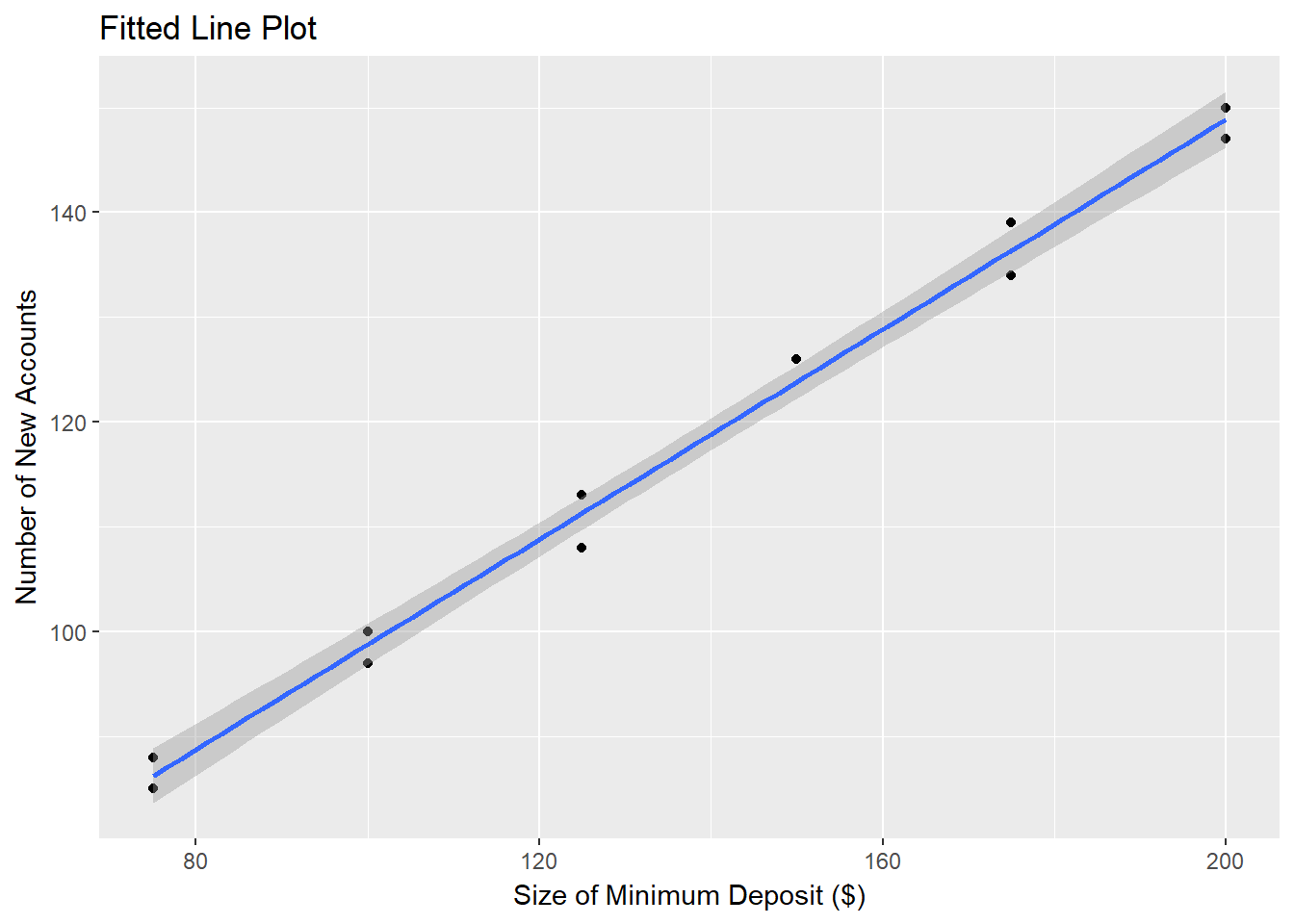Now very little of the total error (SSE = 45.1) is due to lack of a linear fit (SSLF = 6.6). Most of the error is due to random variation in the number of checking accounts (SSPE = 38.5).

pureErrorAnova(lm(New2 ~ Size, data = newaccounts))
## Analysis of Variance Table
##
## Response: New2
##              Df Sum Sq Mean Sq  F value    Pr(>F)
## Size          1 5448.9  5448.9 707.6477 1.403e-06 ***
## Residuals     9   45.1     5.0
##  Lack of fit  4    6.6     1.7   0.2147    0.9194
##  Pure Error   5   38.5     7.7
## ---
## Signif. codes:  0 '***' 0.001 '**' 0.01 '*' 0.05 '.' 0.1 ' ' 1

## 2.11 The Lack of Fit F-test

The F-statistic for the lack of fit F-test is the ratio of the lack of fit mean square MSLF and the pure error mean squre. In the new checking account example with the poor fit, F=14.801 (p=.0056), so reject $$H_0: no lack of fit$$. In the new checking account example with the good fit, F=.2147 (p=.9194), so do not reject $$H_0: no lack of fit$$ (i.e., conclude there is a linear fit).

## 2.12 Further Examples

### Example 1: Highway Sign Reading Distance and Driver Age

Data set signdist is n = 30 observations on driver age (Age) and the maximum distance (Distance) (feet) at which individuals can read a highway sign.

ggplot(signdist, aes(y=Distance, x=Age)) +
geom_point() +
geom_smooth(method = "lm") +
ylab("Distance (feet)") +
xlab("Driver Age") +
ggtitle("Fitted Line Plot")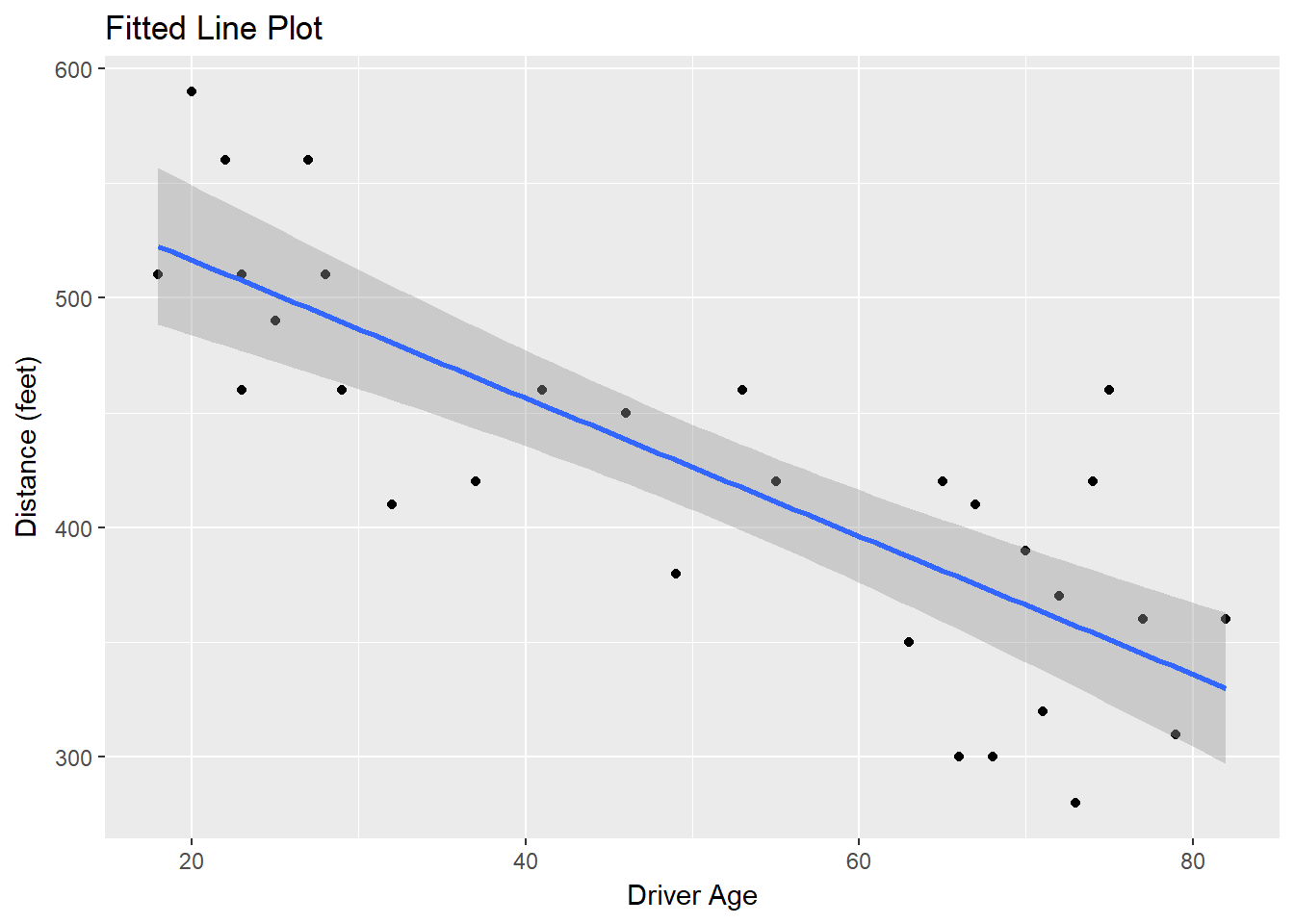The linear model Distance ~ Age produces a parameter estimator Age of $$b_1=-3.0068$$ with SE $$se(b_1)=.4243$$. The test statitic is $$t=b_1/se(b_1)=-7.086$$ which has a p-value of <.0001, so we reject $$H_0: \beta_1=0$$ and conclude there is a linear relationship between distance and age at the $$\alpha=.05$$ level of significance. The 95% confidence interval for $$\beta_1$$ is $$(-3.88, -2.14)$$, meaning the mean sign reading distance decreases between 2.14 and 3.88 feet per each one-year increase in age.

lm_signdist <- lm(Distance ~ Age, data = signdist)
summary(lm_signdist)
##
## Call:
## lm(formula = Distance ~ Age, data = signdist)
##
## Residuals:
##     Min      1Q  Median      3Q     Max
## -78.231 -41.710   7.646  33.552 108.831
##
## Coefficients:
##             Estimate Std. Error t value Pr(>|t|)
## (Intercept) 576.6819    23.4709  24.570  < 2e-16 ***
## Age          -3.0068     0.4243  -7.086 1.04e-07 ***
## ---
## Signif. codes:  0 '***' 0.001 '**' 0.01 '*' 0.05 '.' 0.1 ' ' 1
##
## Residual standard error: 49.76 on 28 degrees of freedom
## Multiple R-squared:  0.642,  Adjusted R-squared:  0.6292
## F-statistic: 50.21 on 1 and 28 DF,  p-value: 1.041e-07
confint.lm(lm_signdist, 2, level = .95)
##         2.5 %   97.5 %
## Age -3.876051 -2.13762

### Example 2: Handspans Data

Data set handheight is n = 167 observations on height (Height) (inches) and hand-span (HandSpan) (inches). Is height linearly related to hand-span?

ggplot(handheight, aes(y=Height, x=HandSpan)) +
geom_point() +
geom_smooth(method = "lm") +
ylab("Height (inches)") +
xlab("Hand-Span (inches)") +
ggtitle("Fitted Line Plot")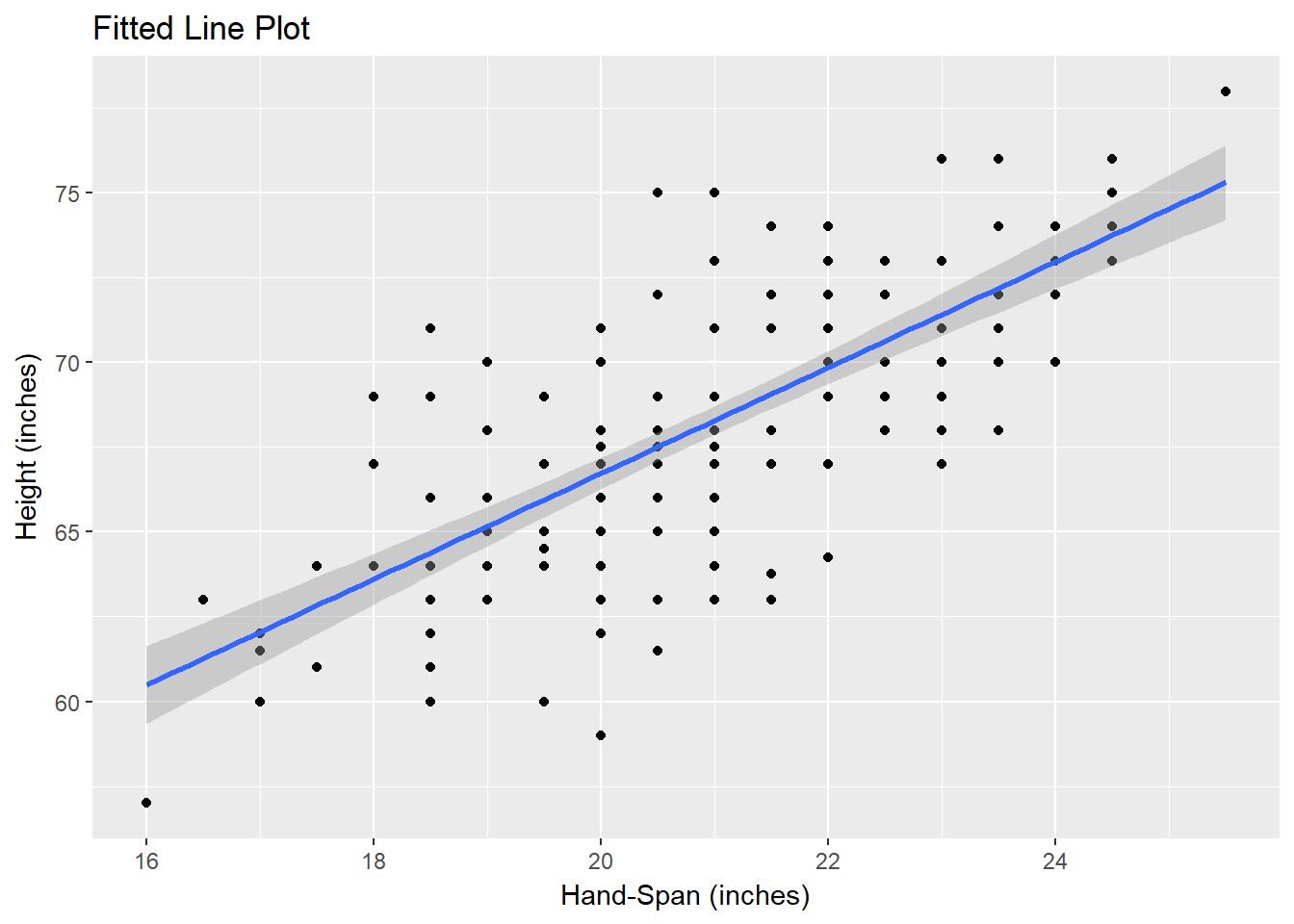In this example, the residual standard error is 2.744. The $$r^2$$ is $$0.5469 = 1500.1 / (1500.1+1242.7)$$. The F-statistic is $$199.2 = 1500.06/7.53$$ (p<.0001, so we reject $$H_0: \beta_1=0$$ and conclude there is a linear relationship between height and hand-spand at the $$\alpha=.05$$ level of significance.

lm_handheight <- lm(Height ~ HandSpan, data = handheight)
summary(lm_handheight)
##
## Call:
## lm(formula = Height ~ HandSpan, data = handheight)
##
## Residuals:
##     Min      1Q  Median      3Q     Max
## -7.7266 -1.7266 -0.1666  1.4933  7.4933
##
## Coefficients:
##             Estimate Std. Error t value Pr(>|t|)
## (Intercept)  35.5250     2.3160   15.34   <2e-16 ***
## HandSpan      1.5601     0.1105   14.11   <2e-16 ***
## ---
## Signif. codes:  0 '***' 0.001 '**' 0.01 '*' 0.05 '.' 0.1 ' ' 1
##
## Residual standard error: 2.744 on 165 degrees of freedom
## Multiple R-squared:  0.5469, Adjusted R-squared:  0.5442
## F-statistic: 199.2 on 1 and 165 DF,  p-value: < 2.2e-16
anova(lm_handheight)
## Analysis of Variance Table
##
## Response: Height
##            Df Sum Sq Mean Sq F value    Pr(>F)
## HandSpan    1 1500.1 1500.06  199.17 < 2.2e-16 ***
## Residuals 165 1242.7    7.53
## ---
## Signif. codes:  0 '***' 0.001 '**' 0.01 '*' 0.05 '.' 0.1 ' ' 1